# Transcendency, measure of

(diff) ← Older revision | Latest revision (diff) | Newer revision → (diff)

transcendence measure

A function characterizing the deviation of a given transcendental number from a set of algebraic numbers of bounded degree and bounded height under a change of bounds on these parameters. For a transcendental numberand natural numbersand, the measure of transcendency is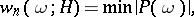where the minimum is taken over all non-zero integer polynomials of degree not exceedingand height not exceeding. It follows from Dirichlet's "box" principle (cf. Dirichlet principle) that the following always holds: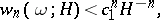where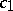depends only on. In many cases it is possible to obtain not only a proof of the transcendency of a numberbut also a lower bound for the measure of transcendency in terms of the degree, and logarithmic or exponential functions ofand. For example, Hermite's method of proof of transcendency ofenables one to obtain the inequality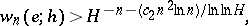whereis an absolute constant and. For any fixedand,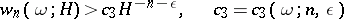for almost-all (in the sense of Lebesgue) real numbers(see Mahler problem). Transcendental numbers can be classified on the basis of the difference in asymptotic behaviour of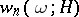under unrestricted variation ofand(see ).

How to Cite This Entry:
Transcendency, measure of. Encyclopedia of Mathematics. URL: http://encyclopediaofmath.org/index.php?title=Transcendency,_measure_of&oldid=17605
This article was adapted from an original article by V.G. Sprindzhuk (originator), which appeared in Encyclopedia of Mathematics - ISBN 1402006098. See original article